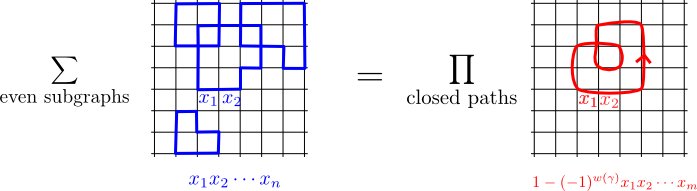## June 29, 2015

### What is a Reedy Category?

#### Posted by Mike ShulmanI’ve just posted the following preprint, which has apparently quite little to do with homotopy type theory.

The notion of Reedy category is common and useful in homotopy theory; but from a category-theoretic point of view it is odd-looking. This paper suggests a category-theoretic understanding of Reedy categories, which I find more satisfying than any other I’ve seen.

Posted at 6:33 PM UTC | Permalink | Followups (20)

### Feynman’s Fabulous Formula

#### Posted by Simon WillertonGuest post by Bruce Bartlett.

There is a beautiful formula at the heart of the Ising model; a formula emblematic of all of quantum field theory. Feynman, the king of diagrammatic expansions, recognized its importance, and distilled it down to the following combinatorial-geometric statement. He didn’t prove it though — but Sherman did.

Feynman’s formula. Let $G$ be a planar finite graph, with each edge $e$ regarded as a formal variable denoted $x_e$. Then the following two polynomials are equal:

$\displaystyle \sum_{H \subseteq_{even} G} x(H) = \prod_{[\vec{\gamma}] \in P(G)} \left(1 - (-1)^{w[\vec{\gamma}]} x[\vec{\gamma}]\right)$I will explain this formula and its history below. Then I’ll explain a beautiful generalization of it to arbitrary finite graphs, expressed in a form given by Cimasoni.

Posted at 7:47 AM UTC | Permalink | Followups (26)

## June 21, 2015

### What’s so HoTT about Formalization?

#### Posted by Mike ShulmanIn my last post I promised to follow up by explaining something about the relationship between homotopy type theory (HoTT) and computer formalization. (I’m getting tired of writing “publicity”, so this will probably be my last post for a while in this vein — for which I expect that some readers will be as grateful as I).

As a potential foundation for mathematics, HoTT/UF is a formal system existing at the same level as set theory (ZFC) and first-order logic: it’s a collection of rules for manipulating syntax, into which we can encode most or all of mathematics. No such formal system requires computer formalization, and conversely any such system can be used for computer formalization. For example, the HoTT Book was intentionally written to make the point that HoTT can be done without a computer, while the Mizar project has formalized huge amounts of mathematics in a ZFC-like system.

Why, then, does HoTT/UF seem so closely connected to computer formalization? Why do the overwhelming majority of publications in HoTT/UF come with computer formalizations, when such is still the exception rather than the rule in mathematics as a whole? And why are so many of the people working on HoTT/UF computer scientists or advocates of computer formalization?

Posted at 6:47 AM UTC | Permalink | Followups (12)

## June 12, 2015

### Carnap and the Invariance of Logical Truth

#### Posted by David CorfieldI see Steve Awodey has a paper just out Carnap and the invariance of logical truth. We briefy discussed this idea in the context of Mautner’s 1946 article back here.

Steve ends the article by portraying homotopy type theory as following in the same tradition, but now where invariance is under homotopy equivalence. I wonder if we’ll see some variant of the model/theory duality he and Forssell found in the case of HoTT.

Posted at 12:09 PM UTC | Permalink | Followups (2)

## June 9, 2015

### Semigroup Puzzles

#### Posted by John BaezSuppose you have a semigroup: that is, a set with an associative product. Also suppose that

$x y x = x$

for all $x$ and all $y$.

Puzzle 1. Prove that

$x y z = x z$

for all $x,y$ and $z$.

Puzzle 2. Prove that

$x x = x$

for all $x$.

Posted at 2:30 PM UTC | Permalink | Followups (43)